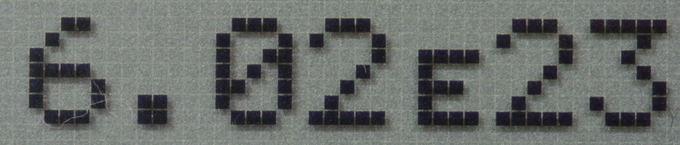## Scientific Notation

#### Learning Objective

• Recognize how to convert between general and scientific notation

#### Key Points

• Scientific notation is expressed in the form $a \times 10^b$ (where “b” is an integer and “a” is any real number), such as $6.02 \times 10^{23}$ .
• Scientific notation allows orders of magnitude to be more easily compared.
• E notation is another form of scientific notation, in which “E” replaces 10, such as 6.02 E 23. This number is the same as $6.02 \times 10^{23}$ .
• Basic operations are carried out in the same manner as with other exponential numbers.

#### Terms

• Order of MagnitudeAn order of magnitude is the class of scale or magnitude of any amount, where each class contains values of a fixed ratio to the class preceding it.
• integerAn element of the infinite and numerable set {…,-3,-2,-1,0,1,2,3,…}.
• Scientific notationA way of writing numbers that are too big or too small to be conveniently written in standard form.

## Scientific Notation

Scientific notation is a more convenient way of writing very small or very large numbers. The general representation for scientific notation is $a \times 10^b$(where “b” is an integer and “a” is any real number). When writing in scientific notation, only include significant figures in the real number, “a.” Significant figures are covered in another section.

To express a number in scientific notation, you move the decimal place to the right if the number is less than zero or to the left if the number is greater than zero.For example, in 456000, the decimal is after the last zero, so to express this in scientific notation, you would need to move the decimal to in between the 4 and 5.

The decimal would move five places to the left to get 4.56 as our $a$ in $a \times 10^b$. The number of times you move the decimal place becomes the integer “b.” In this case, the decimal moved five times. Therefore, our number in scientific notation would be: $4.56 \times 10^5$. Keep in mind that zeroes are not included in “a” because they are not significant figures.

In order to go between scientific notation and decimals, the decimal point is moved the number of spaces indicated by the exponent. A negative exponent tells you to move the decimal point to the right, while a positive exponent tells you to move it to the left.

Examples of scientific notation:

1. 0.0001 = 1 x 10-4
2. .0256 = 2.56 x 10-2
3. 4759000 = 4.759 x 106
4. 5000 = 5 x 103

Another way of writing this expression, as seen on calculators and computer programs, is to use E to represent “times ten to the power of.” An example is shown here:Scientific notation for Avogadro’s numberHere is an example of scientific notation on a calculator. 6.02E23 means the same thing as 6.02 x 1023.

Scientific notation enables comparisons between orders of magnitude. As seen above, scientific notation uses base 10, and if a number is an order of magnitude greater than another, it is 10 times larger. For example, 4.759 x 106 is 3 orders of magnitude bigger than 5 x 103; it is 8 orders of magnitude bigger than 2.56 x 10-2.

## Basic Math with Scientific Notation

Basic operations in scientific notation are carried out in the manner one would carry out exponential functions. Multiplication and division adds or subtracts exponents, respectively. Addition and subtraction require the exponents to be the same. A few examples are shown here: